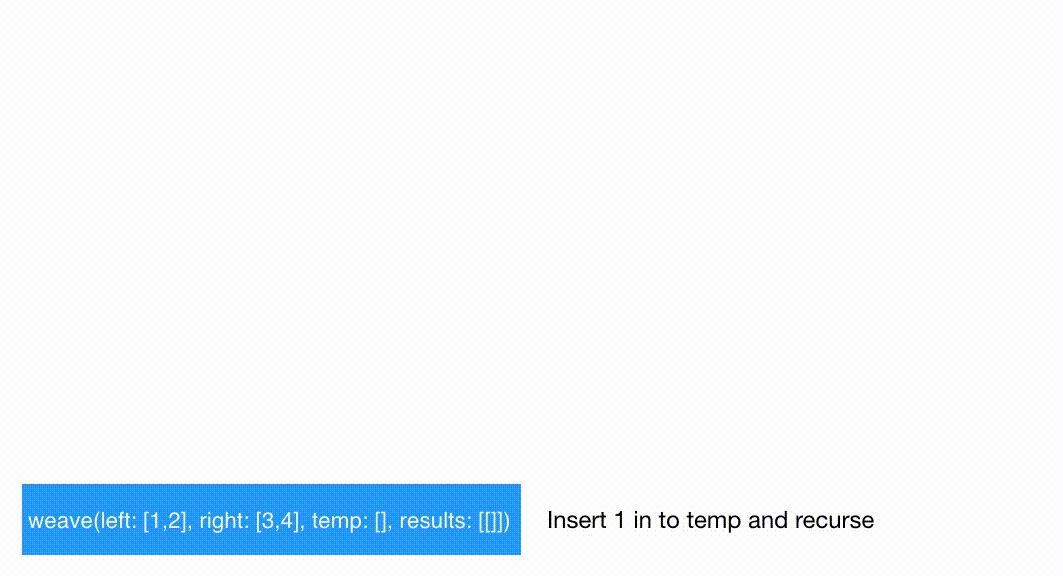This is an interesting recursion problem where the aim is to find all the possible weaves of 2 arrays keeping the relative order of the elements the same.

For example, given two arrays, [1, 2] and [3, 4], the possible solutions are:

• [1, 2, 3, 4]
• [1, 3, 2, 4]
• [1, 3, 4, 2]
• [3, 1, 2, 4]
• [3, 1, 4, 2]
• [3, 4, 1, 2]

As you see above, no matter what, the position of 1 is always before 2 and so is the position of 3 always before 4.

While analyzing this problem, I figured that the solution is pretty similar to the Generate Parentheses problem I solved before. In the parentheses problem, we first check whether we have the option to insert an opening parenthesis, if we do we insert it and carry on the recursion by increasing the counter for number of open parentheses. If we can’t insert an opening parenthesis, we ask ourselves if we can insert a closing paranthesis, if yes, we insert and recurse, if no, we know we have reached one of the solutions.

We’re going to try and use the same approach for this problem too. We will check if an element can be picked from the first array. If yes, pick it and recurse. If no, can we pick from the second array? If yes, pick it and recurse. If no, then we have a possible solution.

To put it in simpler terms, if we’re given two arrays, one of size N and the other of size M, the size of the array after weaving will be (N+M). At each position in the (N+M) sized array, we have a choice, we either pick from the array of size N or from the array of size M. What happens if we have already placed all the elements from one of the arrays in to the weaved array? Simple, just copy the remaining elements from the other array in to the weaved array.

Let’s look at the code.

``````func weave(left: inout [Int], right: inout [Int], prefix: inout [Int], results: inout [[Int]])
{
//1
if left.isEmpty || right.isEmpty {
//2
var result = temp
result.append(contentsOf: left)
result.append(contentsOf: right)
results.append(result)
return
}

//3
let leftFirst = left.removeFirst()
//4
temp.append(leftFirst)
weave(left: &left, right: &right, temp: &temp, results: &results)
//5
temp.removeLast()
//6
left.insert(leftFirst, at: 0)

let rightFirst = right.removeFirst()
temp.append(rightFirst)
weave(left: &left, right: &right, temp: &temp, results: &results)
temp.removeLast()
right.insert(rightFirst, at: 0)
}
``````

And here’s the explanation:

1. This is our base case. Here we check if either of the arrays are empty.
2. If any one (or both) of the arrays is empty, we take the temp parameter and copy it into result. The reason to do so is because we are using inout parameters (pointers in C/C++ world), we do not want to mess up the state of temp when the recursion unrolls.
3. We have the option to pick from the first array. We remove this element from the array.
4. We insert the above element in to the temp array and recurse.
5. We undo our choice, which was above in step 3. The reason for this is because we also want to consider the possibility of picking from the second array.
6. We put the element back in to the original array thereby making sure the input data does not change after this algorithm finishes. The same 6 steps above are followed for the second array.

At the end of the recursion, what you get back is a two dimensional array containing all the possible weaved arrays.

The recursive call stack would look like this:The code is also available as a gist.